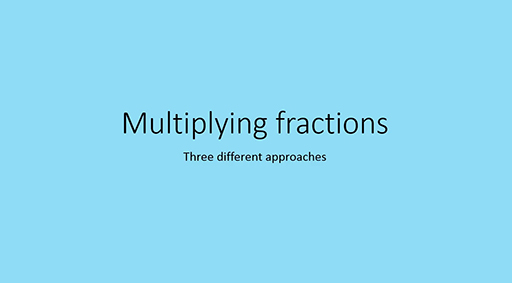Teaching mathematics

Start this free course now. Just create an account and sign in. Enrol and complete the course for a free statement of participation or digital badge if available.

Free course

# 2.4 Multiplying fractions

The procedure for multiplying fractions is straightforward but the conceptual understanding is not so easy to develop.

Watch video 2 on multiplying fractions which demonstrates three different approaches.

Download this video clip.Video player: Video 2 Multiplying fractions using three different approachesSkip transcript: Video 2 Multiplying fractions using three different approaches

#### Transcript: Video 2 Multiplying fractions using three different approaches

INSTRUCTOR:
Multiplying fractions using three different approaches. Suppose we calculate 3/4 times 2/3. Using the procedure of multiplying the two numerators and multiplying the two denominators, we get 6/12, and that cancels to 1/2. Or I could have cancelled numbers top and bottom before multiplying. I can see I've got a 3 on the top and a 3 on the bottom, so I can cancel those 3's. And I've got a multiple of 2 on the top and a multiple 2 on the bottom. 2's into 2 go once, and 2's into 4 go twice. And that leaves me with 1/2.
These procedures seem straightforward, but they don't really build the conceptual understanding which underpins the multiplication of fractions. So let's look at other approaches, which can help build that understanding. The method I'm going to demonstrate now, it helps to remember that in mathematics the word of is interchangeable with multiply. So 3/4 times 2/3 can be thought of as 3/4 of 2/3.
At present, it would be difficult to calculate 3/4 of 2/3. I need to change 2/3 into an equivalent fraction, which is divisible by 4. If I had a numerator of 4, that would be helpful because I can find 3/4 of 4. If I multiply the numerator and denominator in 2/3 by 2, that will give me 4/6, so that will be 3/4 of 4/6. Now 3/4 of 4 is 3, so 3/4 of 4/6 is 3/6, and that's equivalent to a 1/2.
I could display 3/4 times 2/3 as 3/4 of 2/3 on a grid. And a four by three grid would make sense in this case. First, I'm going to shade 3/4 of the grid, so I will shade three out of the four columns. Then, I'm going to shade 2/3, so I will shade two out of the three rows. The overlap is 3/4 of 2/3, and that's 6 out of 12 parts, and that's equivalent to a 1/2.
End transcript: Video 2 Multiplying fractions using three different approaches
Video 2 Multiplying fractions using three different approaches
Interactive feature not available in single page view (see it in standard view).
TM_1